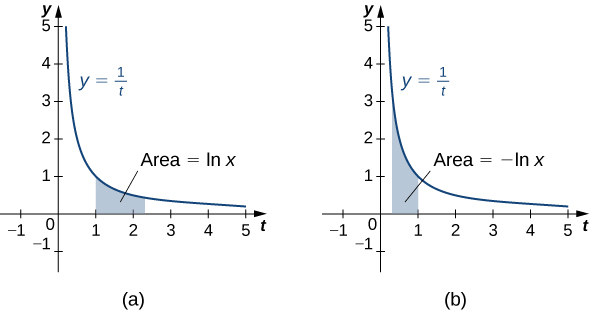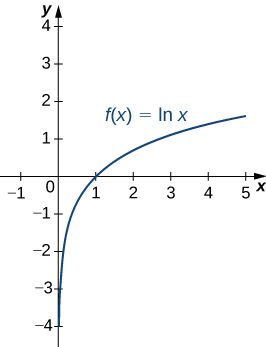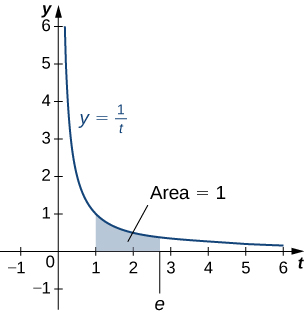## Natural Logarithms

### Learning Outcomes

• Write the definition of the natural logarithm as an integral
• Recognize the derivative of the natural logarithm
• Integrate functions involving the natural logarithmic function
• Define the number 𝑒 through an integral

## The Natural Logarithm as an Integral

Recall the power rule for integrals:

$\displaystyle\int {x}^{n}dx=\frac{{x}^{n+1}}{n+1}+C,n\ne \text{−}1.$

Clearly, this does not work when $n=-1,$ as it would force us to divide by zero. So, what do we do with $\displaystyle\int \frac{1}{x}dx?$ Recall from the Fundamental Theorem of Calculus that ${\displaystyle\int }_{1}^{x}\dfrac{1}{t}dt$ is an antiderivative of $\frac{1}{x}.$ Therefore, we can make the following definition.

### Definition

For $x>0,$ define the natural logarithm function by

$\text{ln}x={\displaystyle\int }_{1}^{x}\frac{1}{t}dt$

For $x>1,$ this is just the area under the curve $y=1\text{/}t$ from 1 to $x.$ For $x<1,$ we have ${\displaystyle\int }_{1}^{x}\frac{1}{t}dt=\text{−}{\displaystyle\int }_{x}^{1}\frac{1}{t}dt,$ so in this case it is the negative of the area under the curve from $x\text{ to }1$ (see the following figure).Figure 1. (a) When $x>1,$ the natural logarithm is the area under the curve $y=\frac{1}{t}$ from $1\text{ to }x.$ (b) When $x<1,$ the natural logarithm is the negative of the area under the curve from $x$ to 1.

Notice that $\text{ln}1=0.$ Furthermore, the function $y=\frac{1}{t}>0$ for $x>0.$ Therefore, by the properties of integrals, it is clear that $\text{ln}x$ is increasing for $x>0.$

## Properties of the Natural Logarithm

Because of the way we defined the natural logarithm, the following differentiation formula falls out immediately as a result of to the Fundamental Theorem of Calculus.

### Derivative of the Natural Logarithm

For $x>0,$ the derivative of the natural logarithm is given by

$\frac{d}{dx}\text{ln}x=\dfrac{1}{x}.$

### Corollary to the Derivative of the Natural Logarithm

The function $\text{ln}x$ is differentiable; therefore, it is continuous.

A graph of $\text{ln}x$ is shown in Figure 2. Notice that it is continuous throughout its domain of $(0,\infty ).$Figure 2. The graph of $f(x)=\text{ln}x$ shows that it is a continuous function.

### Example: Calculating Derivatives of Natural Logarithms

Calculate the following derivatives:

1. $\frac{d}{dx}\text{ln}(5{x}^{3}-2)$
2. $\frac{d}{dx}{(\text{ln}(3x))}^{2}$

### Try It

Calculate the following derivatives:

1. $\frac{d}{dx}\text{ln}(2{x}^{2}+x)$
2. $\frac{d}{dx}{(\text{ln}({x}^{3}))}^{2}$

Watch the following video to see the worked solution to the above Try It.

### Try It

Note that if we use the absolute value function and create a new function $\text{ln}|x|,$ we can extend the domain of the natural logarithm to include $x<0.$ Then $(d\text{/}(dx))\text{ln}|x|=1\text{/}x.$ This gives rise to the familiar integration formula.

### Integral of (1/$u$) du

The natural logarithm is the antiderivative of the function $f(u)=1\text{/}u\text{:}$

$\displaystyle\int \frac{1}{u}du=\text{ln}|u|+C$

### Example: Calculating Integrals Involving Natural Logarithms

Calculate the integral $\displaystyle\int \frac{x}{{x}^{2}+4}dx.$

### Try It

Calculate the integral $\displaystyle\int \frac{{x}^{2}}{{x}^{3}+6}dx.$

Watch the following video to see the worked solution to the above Try It.

### Try It

Although we have called our function a “logarithm,” we have not actually proved that any of the properties of logarithms hold for this function. We do so here.

### Properties of the Natural Logarithm

If $a,b>0$ and $r$ is a rational number, then

1. $\text{ln}1=0$
2. $\text{ln}(ab)=\text{ln}a+\text{ln}b$
3. $\text{ln}(\frac{a}{b})=\text{ln}a-\text{ln}b$
4. $\text{ln}({a}^{r})=r\text{ln}a$

### Proof

1. By definition, $\text{ln}1={\displaystyle\int }_{1}^{1}\frac{1}{t}dt=0.$
2. We have
$\text{ln}(ab)={\displaystyle\int }_{1}^{ab}\frac{1}{t}dt={\displaystyle\int }_{1}^{a}\frac{1}{t}dt+{\displaystyle\int }_{a}^{ab}\frac{1}{t}dt.$

Use $u\text{-substitution}$ on the last integral in this expression. Let $u=t\text{/}a.$ Then $du=(1\text{/}a)dt.$ Furthermore, when $t=a,u=1,$ and when $t=ab,u=b.$ So we get

$\text{ln}(ab)={\displaystyle\int }_{1}^{a}\frac{1}{t}dt+{\displaystyle\int }_{a}^{ab}\frac{1}{t}dt={\displaystyle\int }_{1}^{a}\frac{1}{t}dt+{\displaystyle\int }_{1}^{ab}\frac{a}{t}·\frac{1}{a}dt={\displaystyle\int }_{1}^{a}\frac{1}{t}dt+{\displaystyle\int }_{1}^{b}\frac{1}{u}du=\text{ln}a+\text{ln}b.$

3. Note that
$\frac{d}{dx}\text{ln}({x}^{r})=\frac{r{x}^{r-1}}{{x}^{r}}=\frac{r}{x}.$

Furthermore,

$\frac{d}{dx}(r\text{ln}x)=\frac{r}{x}.$

Since the derivatives of these two functions are the same, by the Fundamental Theorem of Calculus, they must differ by a constant. So we have

$\text{ln}({x}^{r})=r\text{ln}x+C$

for some constant $C.$ Taking $x=1,$ we get

$\begin{array}{ccc}\hfill \text{ln}({1}^{r})& =\hfill & r\text{ln}(1)+C\hfill \\ \hfill 0& =\hfill & r(0)+C\hfill \\ \hfill C& =\hfill & 0.\hfill \end{array}$

Thus $\text{ln}({x}^{r})=r\text{ln}x$ and the proof is complete. Note that we can extend this property to irrational values of $r$ later in this section.
Part iii. follows from parts ii. and iv. and the proof is left to you.

$_\blacksquare$

### Example: Using Properties of Logarithms

Use properties of logarithms to simplify the following expression into a single logarithm:

$\text{ln}9-2\text{ln}3+\text{ln}(\frac{1}{3})$

### Try It

Use properties of logarithms to simplify the following expression into a single logarithm:

$\text{ln}8-\text{ln}2-\text{ln}(\frac{1}{4}).$

## Defining the Number $e$

Now that we have the natural logarithm defined, we can use that function to define the number $e.$

### Definition

The number $e$ is defined to be the real number such that

$\text{ln}e=1$

To put it another way, the area under the curve $y=1\text{/}t$ between $t=1$ and $t=e$ is 1 (Figure 3). The proof that such a number exists and is unique is left to you. (Hint: Use the Intermediate Value Theorem to prove existence and the fact that $\text{ln}x$ is increasing to prove uniqueness.)Figure 3. The area under the curve from 1 to $e$ is equal to one.

The number $e$ can be shown to be irrational, although we won’t do so here (see the activity in Taylor and Maclaurin Series in Calculus 2). Its approximate value is given by

$e\approx 2.71828182846$